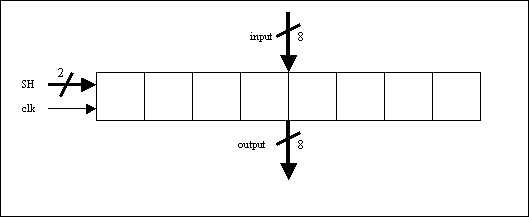SystemC: Synchronous Components

Objectives

• To get familiar with the SystemC, Microsoft Visual C++ 6.0 environment.
• To design synchronous components using SystemC at the Behavioral level.
• Simulate and test components.

Design Problems

For this lab, you are required to write a SystemC description at the behavioral level of a 4-bit up/down counter and a 4-byte register file. Once you have these two components, you need to write a testbench and simulation file to show the correctness of each one. For a general description of registers look in the Embedded Systems Design book on pages 34 and 35.

Up/Down Counter

The inputs, outputs, and functionality of the up/down counter are as follows:

• CLK: This signal will be used as a clock signal.
• PE: Asynchronous enable signal for the counter
• UD: If '0', counter counts down; If '1' counter counts up;
• BD: If '0', counter can count from "0 to 9"; If  '1' counter can count from "0 to 15"
• P: The input of the counter.
• Q: The output of the counter.Size is 4 bytes, i.e. four rows of 8 bits. Each row (byte) is addressable.

The inputs, outputs, and functionality of the parallel load/shift register are as follows:

• clk: This signal will be used as a clock signal.
• input: The 8-bit input.
• output: The 8-bit output.
• SH: The 2-bit load/shift select input.(11 = hold value; 10 = load input; 01 = shift left; 00 = shift right)
• If SH is 01 or 00 it will shift every clock cycle
• When shiftting, move 0 into the register. That is, when the register is left/right shifted eight times, the contents of the register should be 0x00

.